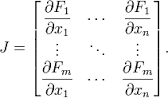# Stability of nonlinear ODE's

## Homework Statement

Find whether this system is stable or unstable at the steady state (x1,x2)=(0,0)
dx1/dt = -x1+2sin(x1)+x2
dx2/dt=2sin(x2)

## Homework Equations## The Attempt at a Solution

z1=x1-0
z2=x2-0
dz1/dt=-z1+z2+2z1
dz2/dt=2z2

Jacobian =
[ 1 1 ]
[ 0 2 ]
so the system is unstable.

This problem is from my notes from class; I'm not 100% certain that it is written correctly. I am very confused about the part where z1 is set equal to x1 (I believe this is called linearization)? Could someone please clarify this step for me and how on earth
dx2/dt=2sin(x2) becomes dz2/dt=2z2 ?

The rest is pretty straightforward.

Dick
Homework Helper

## Homework Statement

Find whether this system is stable or unstable at the steady state (x1,x2)=(0,0)
dx1/dt = -x1+2sin(x1)+x2
dx2/dt=2sin(x2)

## Homework Equations

View attachment 76651

## The Attempt at a Solution

z1=x1-0
z2=x2-0
dz1/dt=-z1+z2+2z1
dz2/dt=2z2

Jacobian =
[ 1 1 ]
[ 0 2 ]
so the system is unstable.

This problem is from my notes from class; I'm not 100% certain that it is written correctly. I am very confused about the part where z1 is set equal to x1 (I believe this is called linearization)? Could someone please clarify this step for me and how on earth
dx2/dt=2sin(x2) becomes dz2/dt=2z2 ?

The rest is pretty straightforward.

If ##z_1=x_1-0## then ##x_1=z_1##. I don't see any problem with that. And the series expansion of ##sin(z)## around ##z=0## is ##z-z^3/3!+z^5/5!+...##. The linearization part is where you ignore the higher powers of ##z## and just replace ##sin(z)## with ##z##, since if ##z## is very close to 0 then the higher powers of ##z## are much smaller than ##z##.

•582153236
If ##z_1=x_1-0## then ##x_1=z_1##. I don't see any problem with that. And the series expansion of ##sin(z)## around ##z=0## is ##z-z^3/3!+z^5/5!+...##. The linearization part is where you ignore the higher powers of ##z## and just replace ##sin(z)## with ##z##, since if ##z## is very close to 0 then the higher powers of ##z## are much smaller than ##z##.
Thanks!
If instead I had dx1/dt=-x13+x2, would it be valid to keep z1 as it is defined now (z1=x1-0) and would dz1/dt=-z13+x2 be true? Since the first term of the series expansion of x3 is x3

Last edited:
Dick